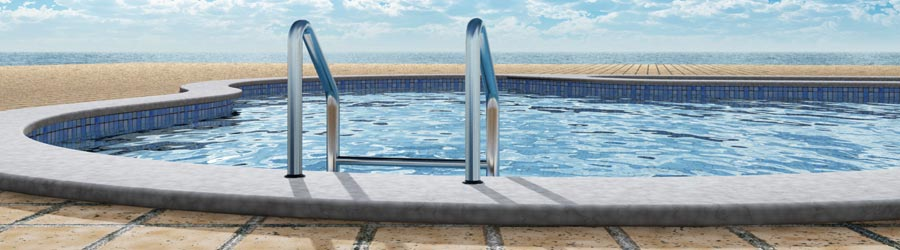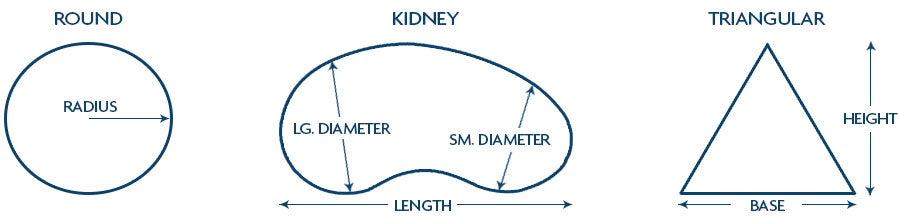# Pool Volume CalculatorTo maintain a safe balance of chemicals in your pool, it is absolutely critical to know the volume of water it holds. Only then can you add the perfect amount of chlorine and other products to keep the water clean.## Square and Rectangular Pools

A square or rectangular pool with one constant depth is easy to measure. Simply multiply the length by the width and then multiply that by the depth. Few pools are shaped so perfectly, though. For a square or rectangular pool with varying depths, you must multiply the length and width, but then multiply that by the average depth. To calculate the average depth of a pool, add together the depth at the deep end and the shallow end and then divide by two.

Keep in mind that a pool is not generally filled all the way to the top. As you calculate the depth or average depth, only measure to the point at which there will be water. If you are a few inches off, it can skew your total.## Round, Kidney and Irregular Pools

If your pool is round or circular, multiply the radius (one-half of the length across the center point) by itself (radius squared). Multiply that total by the average depth of the pool, then multiply by 3.14 (pi).

For more irregular shapes, you will need to split the pool into sections, calculate the surface area of each section, and then add them together. For example, for a kidney-shaped pool, you can calculate the surface area of a rectangular section in the center and then treat the two semicircles at the ends as one circle. Once you have done that, multiply the total surface area by the average depth. With irregular shapes, you have to get creative, but our Pool Water Volume Calculator can save you a great deal of trouble.

### Converting to Gallons

When you add chlorine, you will need to know how many gallons of water your pool holds. That is easy to determine once you know your pool's volume in cubic feet. There are 7.5 gallons of water in one cubic foot, so after you have figured out the number of cubic feet, multiply it by 7.5 to determine how many gallons of water are in your pool. Then, add products accordingly.